List Processing List Comprehension List comprehension is a

• Slides: 13List Processing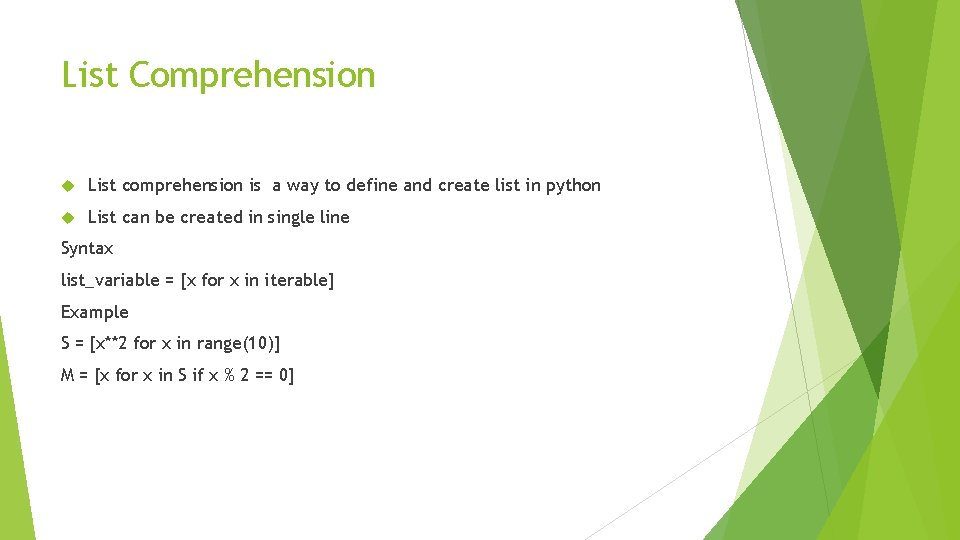List Comprehension List comprehension is a way to define and create list in python List can be created in single line Syntax list_variable = [x for x in iterable] Example S = [x**2 for x in range(10)] M = [x for x in S if x % 2 == 0]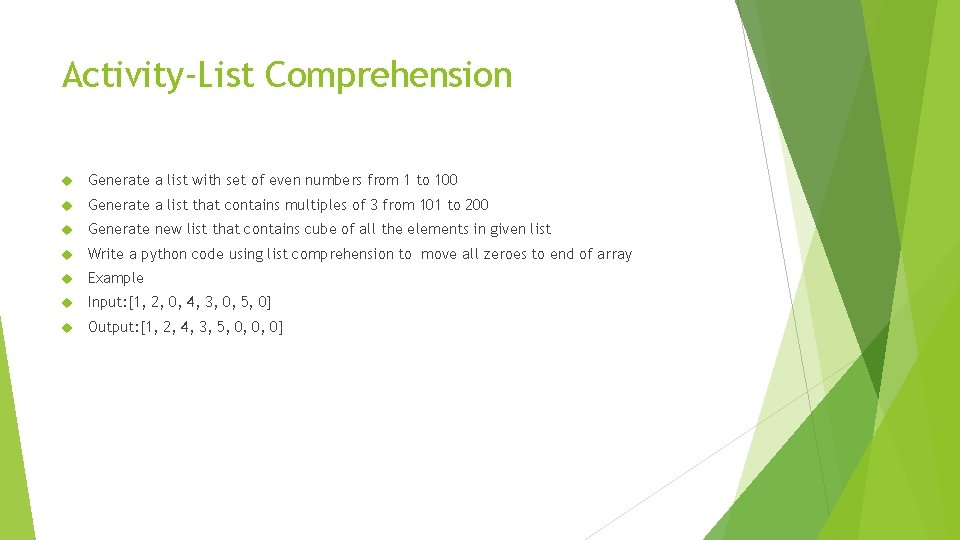Activity-List Comprehension Generate a list with set of even numbers from 1 to 100 Generate a list that contains multiples of 3 from 101 to 200 Generate new list that contains cube of all the elements in given list Write a python code using list comprehension to move all zeroes to end of array Example Input: [1, 2, 0, 4, 3, 0, 5, 0] Output: [1, 2, 4, 3, 5, 0, 0, 0]Searching Linear Search Binary SearchLinear Search Function def search(arr, x): for i in range(len(arr)): if arr[i] == x: return i return -1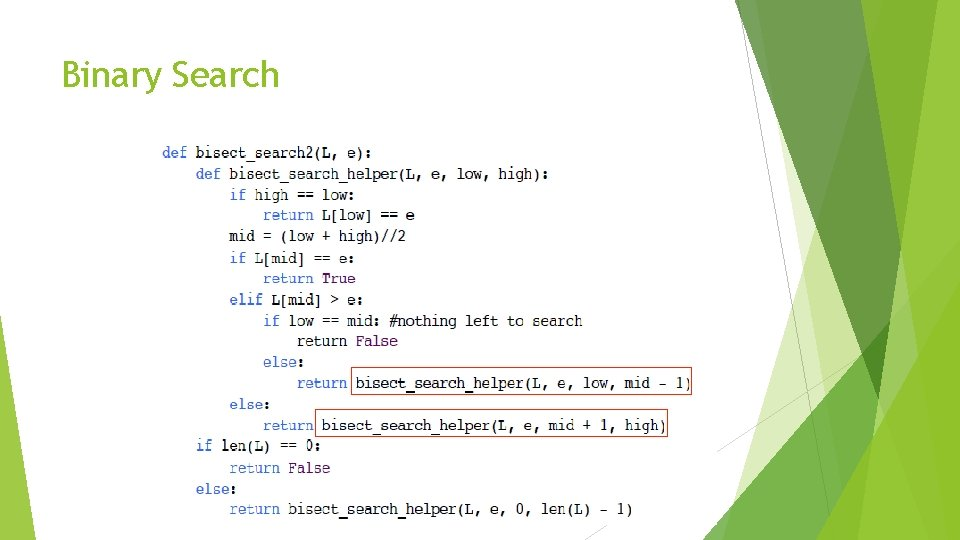Binary SearchSorting Bubble sort Selection sortL 1 = [30, 45, 20, 90, 78] Iteration 1: 30 40 45 20 90 78 No Exchange 30 40 45 20 90 78 Exchange 30 40 20 45 No Exchange 90 78 30 40 45 20 78 90 Exchange After first Iteration, still the output is not in the sorted order. Repeat the above steps for iteration 2L 1 = [30, 40, 20, 45, 78, 90] –After Iteration 1 Iteration 2: Apply the bubble sort to the output of the first iteration 30 40 20 45 78 90 No Exchange 30 40 20 45 78 90 Exchange 30 20 40 45 78 90 No Exchange 30 40 45 20 90 78 After second iteration still the output is not in a sorted order. Repeat the above steps for iteration 3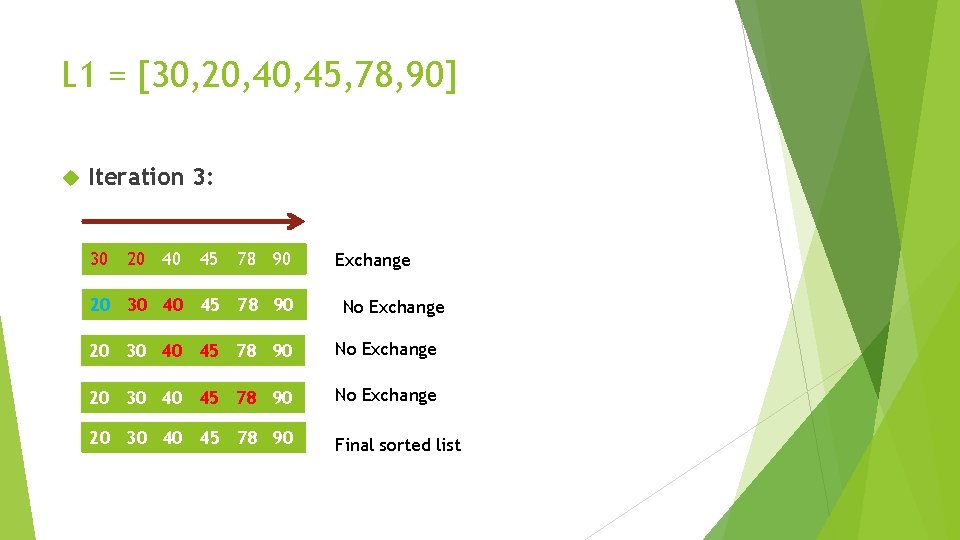L 1 = [30, 20, 45, 78, 90] Iteration 3: 30 20 40 45 78 90 20 30 40 45 78 90 Exchange No Exchange 20 30 40 45 78 90 Final sorted listBubble sort def bubble. Sort(arr): n = len(arr) for i in range(n): for j in range(i+1, n): if arr[i] > arr[j] : arr[i], arr[j] = arr[j], arr[i] # Driver code to test above arr = [64, 34, 25, 12, 22, 11, 90] bubble. Sort(arr) print ("Sorted array is: ") for i in range(len(arr)): print (arr[i])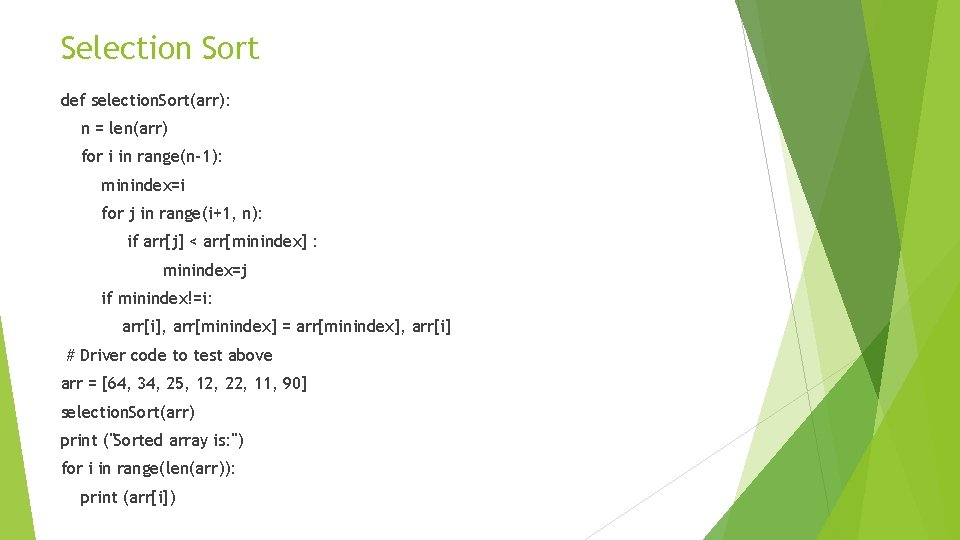Selection Sort def selection. Sort(arr): n = len(arr) for i in range(n-1): minindex=i for j in range(i+1, n): if arr[j] < arr[minindex] : minindex=j if minindex!=i: arr[i], arr[minindex] = arr[minindex], arr[i] # Driver code to test above arr = [64, 34, 25, 12, 22, 11, 90] selection. Sort(arr) print ("Sorted array is: ") for i in range(len(arr)): print (arr[i])Thank You